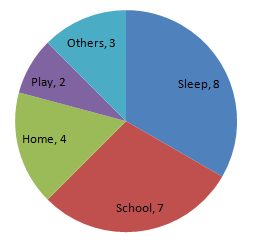# The number of hours, spent by a school boy on different activities in a working day, is given below:

Question:

The number of hours, spent by a school boy on different activities in a working day, is given below:

 Activities Sleep School Home Play Others Total Number of hours 8 7 4 2 3 24

Present the information in the form of a pie-chart.

Solution:

We know:

Central angle of a component = (component value / sum of component values×">× 360)

Here, total number of hours = 24

Thus, the central angle for each component can be calculated as follows:

 Activity Number of hours Sector angle Sleep 8 8/24 ×">× 360 = 120o School 7 7/24    ×">× 360 = 105o Home 4 4/24 ×">× 360 = 60o Play 2 2/24 ×">× 360 = 30o Others 3 3/24 ×">× 360 = 45o

Now, the pie chat that represents the given data can be constructed by following the steps given below:

Step 1 : Draw a circle of an appropriate radius.

Step 2 : Draw a vertical radius of the circle drawn in step 1.

Step 3 : Choose the largest central angle. Here, the largest central angle is 120o. Draw a sector with the central angle 120o in such a way that one of its radii coincides with the radius drawn in step 2 and another radius is in its counter-clockwise direction.

Step 4 : Construct other sectors representing other items in the clockwise direction in descending order of magnitudes of their central angles.

​Step 5 : Shade the sectors with different colours and label them as shown as in the figure below.​​